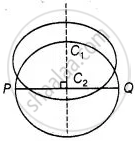# If a number of circles pass through the end points P and Q of a line segment PQ, then their centres lie on the perpendicular bisector of PQ. - Mathematics

MCQ
True or False

If a number of circles pass through the endpoints P and Q of a line segment PQ, then their centres lie on the perpendicular bisector of PQ.

• True

• False

#### Solution

This statement is True.

Explanation:We draw two circle with centre C1 and C2 passing  through the endpoint P and Q at a line segment PQ.

We know, that perpendicular bisectors of a chord of a circle always passes through the centre of circle.

Thus, perpendicular bisector of PQ passes through C1 and C2.

Similarly, all the circle passing through PQ will have their centre on perpendiculars bisectors of PQ.

Concept: Concept of Circle - Centre, Radius, Diameter, Arc, Sector, Chord, Segment, Semicircle, Circumference, Interior and Exterior, Concentric Circles
Is there an error in this question or solution?

#### APPEARS IN

NCERT Mathematics Exemplar Class 10
Chapter 9 Circles
Exercise 9.2 | Q 9 | Page 106
Share